# Entropy Activity

-Introduction

-The reaction coordinate

-The energy landscape

-Population distributions

-Motion at constant temperature, and exchange of energy with the heat bath

-Thermally activated processes

-Energy and temperature determine the populations

-Entropy and free energy

-Mathematical derivation of free energy

 < Previous | Next >

## Mathematical derivation of free energy

On the previous page, we used the expression: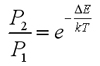for the ratio of populations between two states. This ratio holds between any two specific states, such as individual platforms for the bouncing balls, or individual sides on which the box can stand: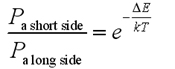(A box is standing up if it is standing on a short side of the rectangular box, and is lying down if it is standing on a long side.)

We will refer to these specific states (a box standing on a specific side) as configurations. Now suppose that we want to group configurations together into states such as "box standing up" and "box lying down". For the three-dimensional box of the previous section, four configurations are grouped into the "box standing up" state and two configurations are grouped into the "box lying down" state. The total population of boxes standing up is a sum of the populations in the four standing-up configurations, and since these states have equal energy, they have equal populations:

Pall standing-up configurations = Pshort side 1 + Pshort side 2 + Pshort side 3 + Pshort side 4 = 4 * Pa short side

Similarly, the total population of boxes lying down is:

Pall lying down configurations = Plong side 1 + Plong side 2 = 2 * Pa long side

The ratio is then: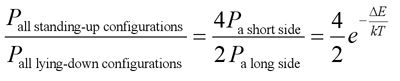More generally, we can write that the population between two states is: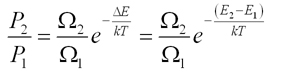where Ωn is the number of configurations grouped together to make the state.

For our box example, Ω2=4 (the number of ways the box can stand up) and Ω2 =2 (the number of ways the box can lie down).

Question: Consider a box with dimensions as shown below. Suppose the difference in energy between the box standing up and laying down is ΔE. What is the ratio between the total pollution of boxes standing up versus laying down, Pstanding up/Playing down, at a given temperature T?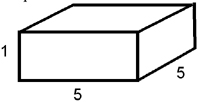We can get from the expression: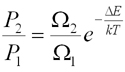to free energy through the following mathematical manipulations. First, we re-write the above ratio as: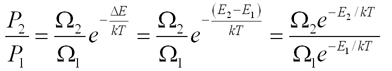If we want the effects of Ω to be comparable to the effects of energy, we need to move Ω into the exponential. Since ln and exp cancel one another, we can write:

Ω = elnΩ

We also want to have kT in the denominator of the exponent, so we multiply and divide by kT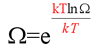If we define entropy S as k lnΩ, then the above becomes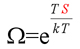We can now put this back into the population ratio to get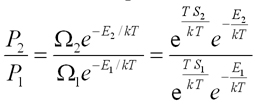Combining the exponentials together (recall that exey = ex+y),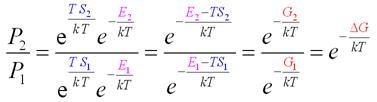Where we have used G = E - TS. This leads us to the result we introduced at the end of the previous section: when you group configurations together into states, the energy should be replaced with the free energy. It also gives us a molecular-level definition of entropy: S = -k lnΩ, the formula inscribed on Boltzmann tombstone (Boltzmann rightly considered this expression the ultimate achievement of his life's work, the ability to connect the entropy measured in thermodynamic experiments on macroscopic systems to the atomic/molecular world.)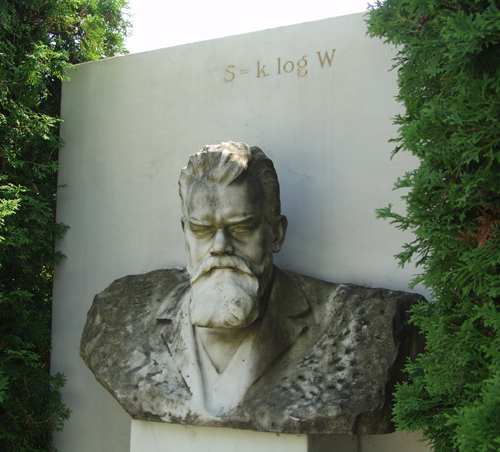Vienna, Austria. Boltzmann's Tomb in the Zentralfriedhof (Central Cemetery).
Photo © Tom Schneider

 < Previous | Next >